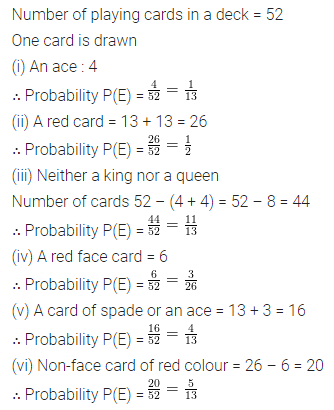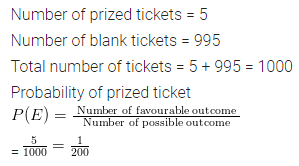# ML Aggarwal Class 8 Solutions for ICSE Maths Chapter 19 Data Handling Ex 19.3

## ML Aggarwal Class 8 Solutions for ICSE Maths Chapter 19 Data Handling Ex 19.3

Question 1.
List the outcomes you can see in these experiments.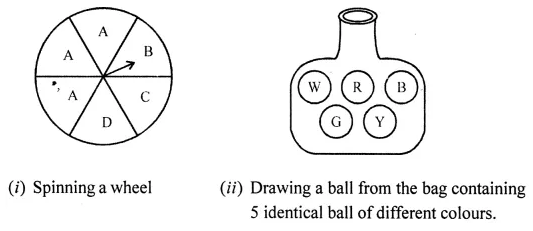Solution:Question 2.
A die is rolled once. Find the probability of getting
(i) an even number
(ii) a multiple of 3
(iii) not a multiple of 3
Solution: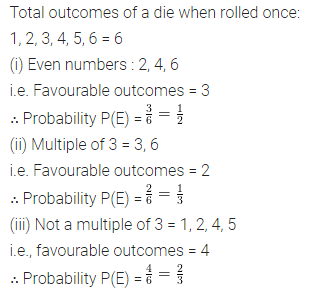Question 3.
Two coins are tossed together. Find the probability of getting
(i) two tails
(ii) atleast one tail
(iii) no tail
Solution: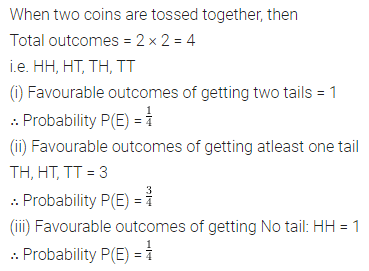Question 4.
Three coins are tossed together. Find the probability of getting
(ii) atleast one tail
(iii) atmost one tail.
Solution:Question 5.
Two dice are rolled simultaneously. Find the probability of getting
(i) the sum as 7
(ii) the sum as 3 or 4
(iii) prime numbers on both the dice.
Solution: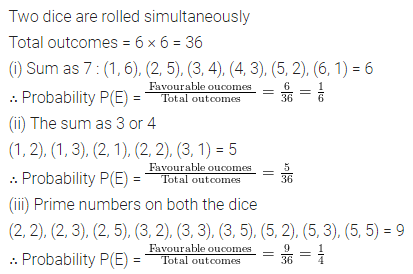Question 6.
A fcox contains 600 screws, one tenth are rusted. One screw is taken out at random from the box. Find the probability that it is
(i) a rusted screw
(ii) not a rusted screw
Solution: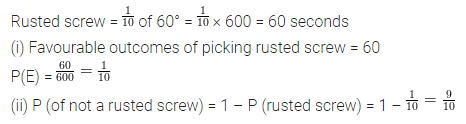Question 7.
A letter is chosen from the word ‘TRIANGLE’. What is the probability that it is a vowel?
Solution: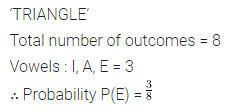Question 8.
A bag contains 5 red, 6 black and 4 white balls. A ball is drawn at random from the bag, find the probability the ball is drawn is
(i) white
(ii) not black
(iii) red or black
(iv) neither red nor black
Solution: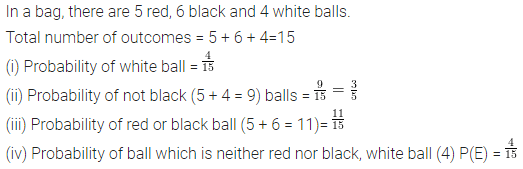Question 9.
A box contains 17 cards numbered 1, 2, 3, ……….,17 and are mixed thoroughly. A card is drawn at random from the box. Find the probability that the number on the card is
(i) odd
(ii) even
(iii) prime
(iv) divisible by 3
(v) divisible by 2 and 3 both
Solution:Question 10.
A card is drawn from a well-shuffled pack of 52 cards. Find the probability that the card drawn is:
(i) an ace
(ii) a red card
(iii) neither a king nor a queen
(iv) a red face card or an ace